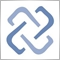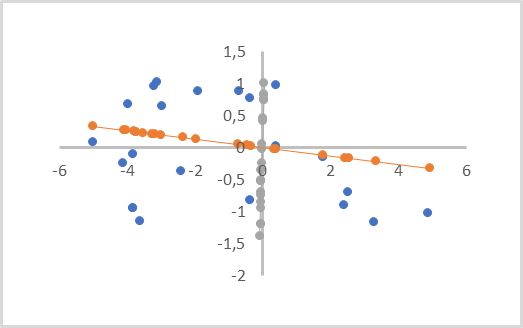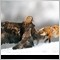# Discussion of article "Neural networks made easy (Part 17): Dimensionality reduction"Moderator
251607

New article Neural networks made easy (Part 17): Dimensionality reduction has been published:

In this part we continue discussing Artificial Intelligence models. Namely, we study unsupervised learning algorithms. We have already discussed one of the clustering algorithms. In this article, I am sharing a variant of solving problems related to dimensionality reduction.

Principal component analysis was invented by the English mathematician Karl Pearson in 1901. Since then, it has been successfully used in many science fields.

To understand the essence of the method, I propose to take the simplified task related to the reducing the dimension of a two-dimensional data array to a vector. From a geometric point of view, this can be represented as a projection of points of a plane onto a straight line.

In the figure below, the initial data is represented by blue dots. There are two projections, on the orange and gray lines, with dots of the corresponding color. As you can see, the average distance from the initial points to their orange projections is smaller than the similar distances to the gray projections. Gray projections have overlapping of projections of points. So, the orange projection is more preferable, as it separates all individual points and loses less data when reducing the dimension (distance from points to their projections).

Such a line is called the principal component. That is why the method is called Principal Component Analysis.

From a mathematical point of view, each principal component is a numerical vector which size is equal to the dimension of the original data. The product of the vector of original data describing one system state by the corresponding vector of the principal component generates the projection point of the analyzed state on the straight line.

Depending on the original data dimension and the requirements for dimensionality reduction, there can be several principal components, but no more than the original data dimension. When rendering a volumetric projection, there will be three of them. When compressing data, the allowable error is usually a loss of up to 1% of data.Visually this looks similar to a linear regression. But these are completely different methods, and they produce different results.

Author: Dmitriy Gizlyk2098

Always heard Neurals were the future of AI.